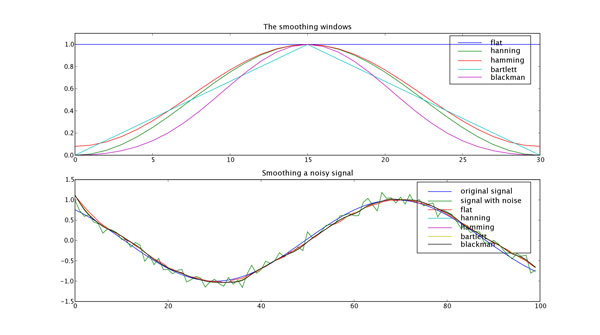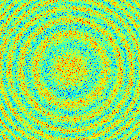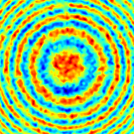This is an archival dump of old wiki content --- see scipy.org for current material.

# Smoothing of a 1D signal

This method is based on the convolution of a scaled window with the signal. The signal is prepared by introducing reflected window-length copies of the signal at both ends so that boundary effect are minimized in the beginning and end part of the output signal.

## Code

```   1 import numpy
2
3 def smooth(x,window_len=11,window='hanning'):
4     """smooth the data using a window with requested size.
5
6     This method is based on the convolution of a scaled window with the signal.
7     The signal is prepared by introducing reflected copies of the signal
8     (with the window size) in both ends so that transient parts are minimized
9     in the begining and end part of the output signal.
10
11     input:
12         x: the input signal
13         window_len: the dimension of the smoothing window; should be an odd integer
14         window: the type of window from 'flat', 'hanning', 'hamming', 'bartlett', 'blackman'
15             flat window will produce a moving average smoothing.
16
17     output:
18         the smoothed signal
19
20     example:
21
22     t=linspace(-2,2,0.1)
23     x=sin(t)+randn(len(t))*0.1
24     y=smooth(x)
25
27
28     numpy.hanning, numpy.hamming, numpy.bartlett, numpy.blackman, numpy.convolve
29     scipy.signal.lfilter
30
31     TODO: the window parameter could be the window itself if an array instead of a string
32     NOTE: length(output) != length(input), to correct this: return y[(window_len/2-1):-(window_len/2)] instead of just y.
33     """
34
35     if x.ndim != 1:
36         raise ValueError, "smooth only accepts 1 dimension arrays."
37
38     if x.size < window_len:
39         raise ValueError, "Input vector needs to be bigger than window size."
40
41
42     if window_len<3:
43         return x
44
45
46     if not window in ['flat', 'hanning', 'hamming', 'bartlett', 'blackman']:
47         raise ValueError, "Window is on of 'flat', 'hanning', 'hamming', 'bartlett', 'blackman'"
48
49
50     s=numpy.r_[x[window_len-1:0:-1],x,x[-1:-window_len:-1]]
51     #print(len(s))
52     if window == 'flat': #moving average
53         w=numpy.ones(window_len,'d')
54     else:
55         w=eval('numpy.'+window+'(window_len)')
56
57     y=numpy.convolve(w/w.sum(),s,mode='valid')
58     return y
59
60
61
62
63 from numpy import *
64 from pylab import *
65
66 def smooth_demo():
67
68     t=linspace(-4,4,100)
69     x=sin(t)
70     xn=x+randn(len(t))*0.1
71     y=smooth(x)
72
73     ws=31
74
75     subplot(211)
76     plot(ones(ws))
77
78     windows=['flat', 'hanning', 'hamming', 'bartlett', 'blackman']
79
80     hold(True)
81     for w in windows[1:]:
82         eval('plot('+w+'(ws) )')
83
84     axis([0,30,0,1.1])
85
86     legend(windows)
87     title("The smoothing windows")
88     subplot(212)
89     plot(x)
90     plot(xn)
91     for w in windows:
92         plot(smooth(xn,10,w))
93     l=['original signal', 'signal with noise']
94     l.extend(windows)
95
96     legend(l)
97     title("Smoothing a noisy signal")
98     show()
99
100
101 if __name__=='__main__':
102     smooth_demo()
```

## Figure# Smoothing of a 2D signal

Convolving a noisy image with a gaussian kernel (or any bell-shaped curve) blurs the noise out and leaves the low-frequency details of the image standing out.

## Functions used

```   1 def gauss_kern(size, sizey=None):
2     """ Returns a normalized 2D gauss kernel array for convolutions """
3     size = int(size)
4     if not sizey:
5         sizey = size
6     else:
7         sizey = int(sizey)
8     x, y = mgrid[-size:size+1, -sizey:sizey+1]
9     g = exp(-(x**2/float(size)+y**2/float(sizey)))
10     return g / g.sum()
11
12 def blur_image(im, n, ny=None) :
13     """ blurs the image by convolving with a gaussian kernel of typical
14         size n. The optional keyword argument ny allows for a different
15         size in the y direction.
16     """
17     g = gauss_kern(n, sizey=ny)
18     improc = signal.convolve(im,g, mode='valid')
19     return(improc)
```

## Example

```from scipy import *

X, Y = mgrid[-70:70, -70:70]
Z = cos((X**2+Y**2)/200.)+ random.normal(size=X.shape)
``````blur_image(Z, 3)
```The attachment cookb_signalsmooth.py contains a version of this script with some stylistic cleanup.